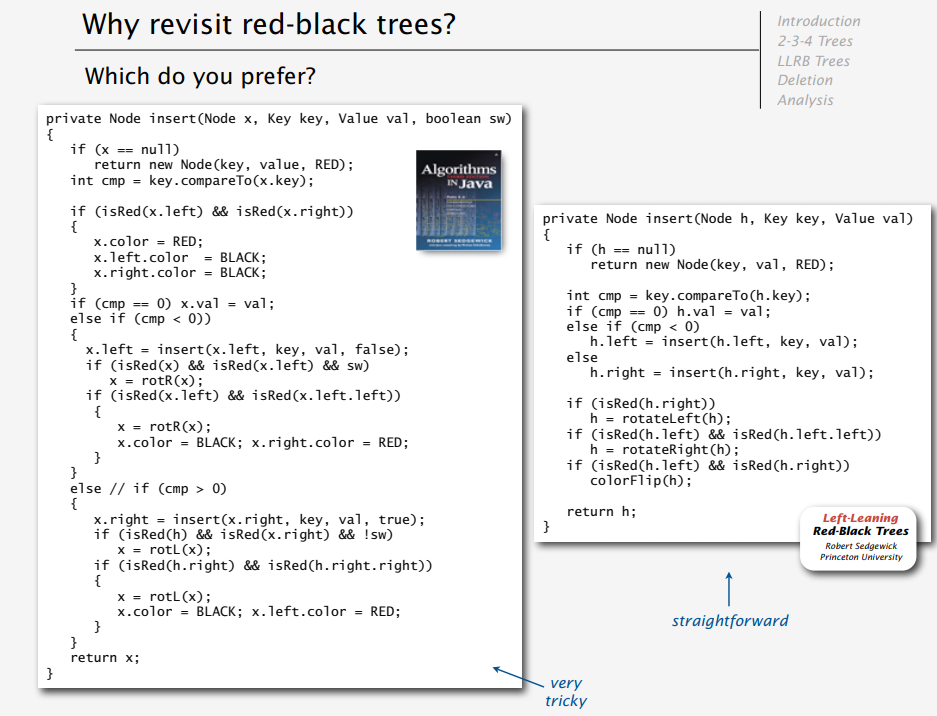## 0x01 RSA

RSA目前是应用最广泛的加密技术之一

RSA的生成步骤如下：

1. 随意选择两个大质数p q，并计算$N=p*q$
2. $r=(p-1)(q-1)$ 这是根据欧拉函数$r = φ(N) = φ(p)\cdotφ(q) = (p-1)\cdot(q-1)$获得的
3. 选择一个小于r并与r互质的整数e（通常取65537），求得e关于r的模反元素d（$ed = 1(mod r)$，模反元素存在，当且仅当e与r互质）
4. 销毁p和q
5. (N , e)是公钥，(N, d)为私钥

• 加密过程： $m^e = c ,(\mod n ,)$
• 解密过程： $c^d = m ,(\mod n ,)$

## 0x02 KMP算法

KMP是字符串匹配的算法，由普通的O(MN)缩减到了O(M+N)。KMP 算法的意思是找到目标字符串（模式串）中已经匹配过的前缀，并略去其匹配过程。

## 0x03 AVL树与红黑树

• AVL树：最早的平衡二叉树之一，应用比较少。Windows对进程地址空间的管理用到了AVL树。
• 红黑树：广泛应用在C++的STL中，比如map和set都是红黑树实现的
• B/B+树：磁盘文件组织，数据库的索引
• Trie树：统计排序大量字符串（自动机）

#### 1. AVL树（平衡二叉树）

AVL树的左子树和右子树的深度之差(平衡因子)的绝对值不超过1，且它的左子树和右子树都是一颗平衡二叉树。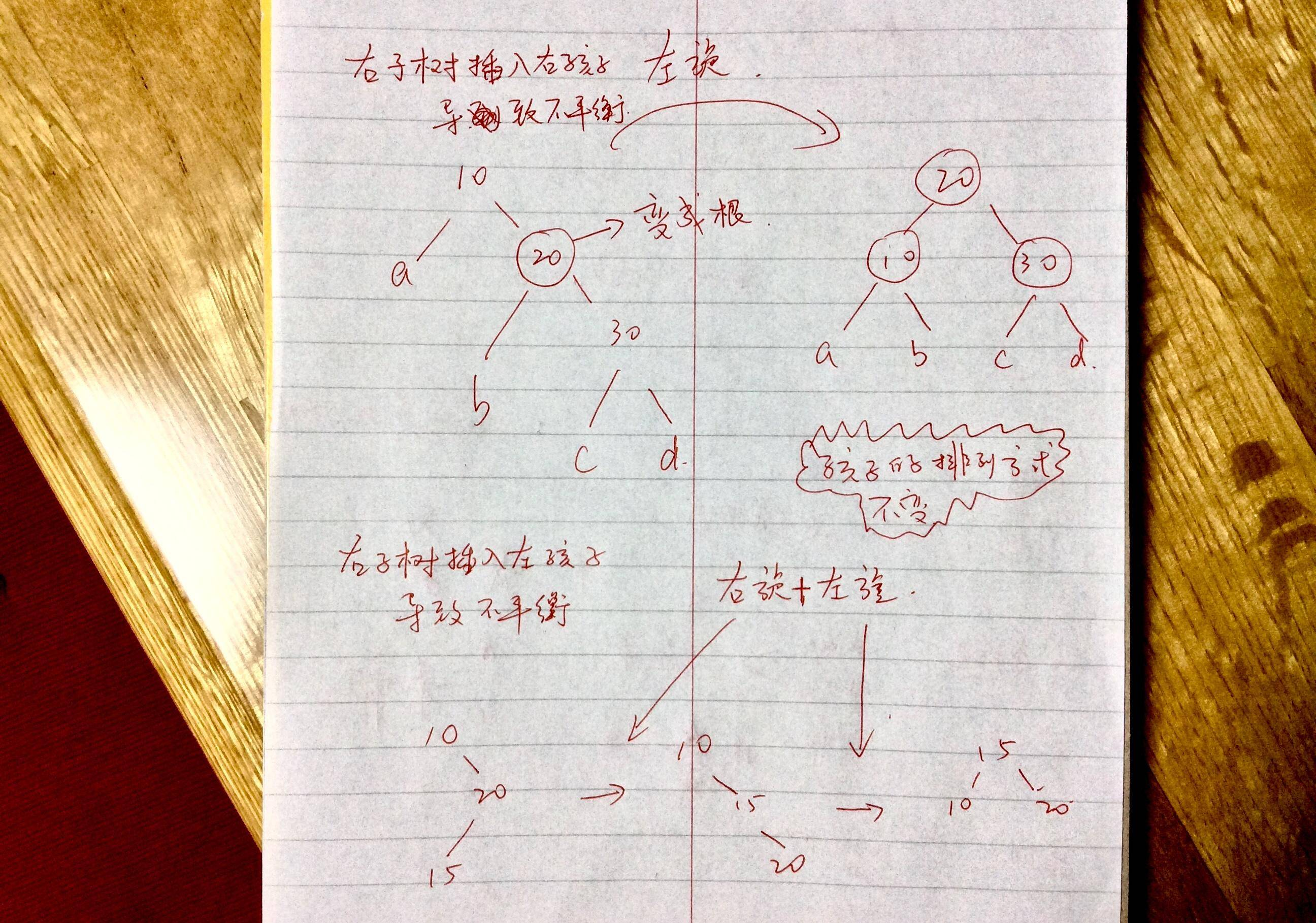#### 2. 红黑树

下图出自"程序员小灰"公众号1. 每个节点不是红色就是黑色的；
2. 根节点总是黑色的；
3. 如果节点是红色的，则它的子节点必须是黑色的（反之不一定）；
4. 从根节点到叶节点或空子节点的每条路径，必须包含相同数目的黑色节点（即相同的黑色高度）。

• 2节点：瘦节点
• 3节点：胖节点
• 4节点：胖胖节点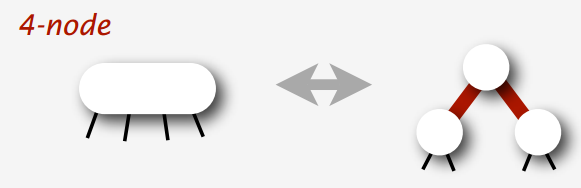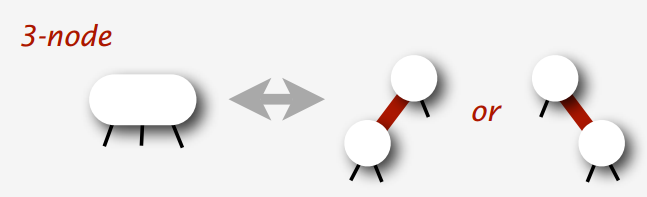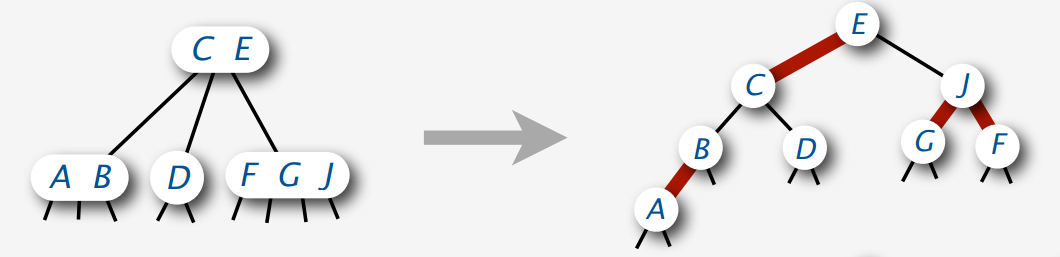1. 当前节点N是树中的根节点—>将节点直接染成黑色
2. 当前节点的父亲P是黑色—>直接添加
3. 算法导论 情况1叔叔是红色（相当与出现了5节点）—>父亲，叔叔，爷爷全部变色
4. 算法导论 情况2,3叔叔是黑色（出现了胖胖节点，变成其标准形状）—>将N变为左孩子（左旋），并对整颗树进行右旋。形成胖胖节点的标准形状

#### 3. 左倾红黑树

1. 插入红节点，如果如果右倾，左旋为左倾
2. 如果出现连续的红节点，右旋转换成胖胖节点
3. 出现了胖胖节点，立刻分解成3个瘦节点（变色）